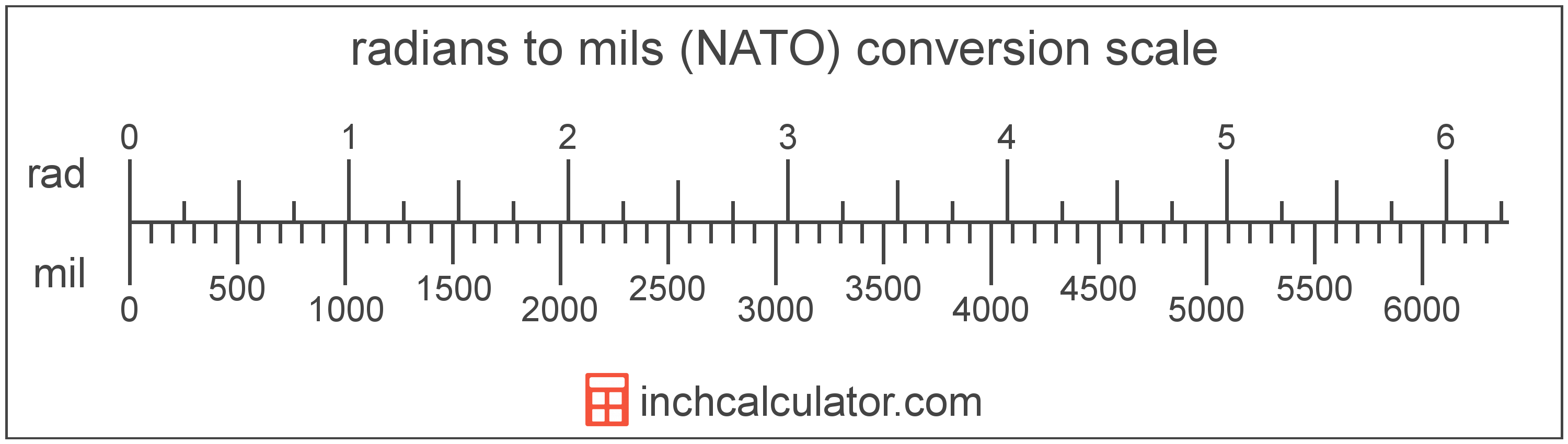# Radians to Mils (NATO) Converter

Enter the angle in radians below to get the value converted to mils. The calculator supports values containing decimals, fractions, and π: (π/2, 1/2π, etc)

Results in Mils:Do you want to convert mils to radians?

## How to Convert Radians to Mils

To convert a measurement in radians to a measurement in mils, multiply the angle by the following conversion ratio: 1,018.591636 mils/radian.

Since one radian is equal to 1,018.591636 mils, you can use this simple formula to convert:

The angle in mils is equal to the angle in radians multiplied by 1,018.591636.

For example, here's how to convert 5 radians to mils using the formula above.
mils = (5 rad × 1,018.591636) = 5,092.958179 mil### How Many Mils Are in a Radian?

There are 1,018.591636 mils in a radian, which is why we use this value in the formula above.

A radian is the measurement of angle equal to the length of an arc divided by the radius of the circle or arc. 1 radian is equal to 180/π degrees, or about 57.29578°. There are about 6.28318 radians in a circle.

The radian is the SI derived unit for angle in the metric system. Radians can be abbreviated as rad, and are also sometimes abbreviated as c, r, or R. For example, 1 radian can be written as 1 rad, 1 c, 1 r, or 1 R.

Radians are often expressed using their definition. The formula to find an angle in radians is θ = s/r, where the angle in radians θ is equal to the arc length s divided by the radius r. Thus, radians may also be expressed as the formula of arc length over the radius.

Radians are also considered to be a "unitless" unit. That is, when multiplying or dividing by radians, the result does not include radians as part of the final units.

For example, when determining the length of an arc for a given angle, we use the formula above, rearranged to be s = θr. If θ is in radians and r is in meters, then the units of s will be meters, not radian-meters. If θ were in degrees, however, then s would have units of degree-meters.

## What Is a Mil?

A mil, short for milliradian, is equal to 1/6,400 of a circle. The mil used by the US military and NATO forces is slightly different than the true value of a milliradian, which is equal to 1/6,283 of a circle.

During World War I the US adopted what is now the NATO mil to replace degrees and minutes for use in artillery sights. They opted to round mils to 6,400 per circle for simplicity at the time. Today, the mil is commonly used to measure adjustment of sights and scopes of firearms.

There is rightfully much confusion as a result of the mil adopted by the US military and NATO being slightly different than the milliradian.

A mil is sometimes also referred to as an angular mil. Mils can be abbreviated as mil; for example, 1 mil can be written as 1 mil.

## Radian to Mil Conversion Table

Table showing various radian measurements converted to mils.